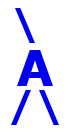## AIRES library reference: nuclcode.

```c
c     Routine directly callable from C/C++ (it could be necessary to
c     append an underscore, "_", to its name depending on the C/C++
c     compiler used).
c
c
function nuclcode(z, n, irc)
c
c     Returning the code corresponding to a nucleus of charge z and
c     n neutrons.
c     The formula used for encoding is:
c
c               nuclcode = (M0 + aa) + (M - 1) z + n
c
c     where M0, M and aa are constants adequately chosen.
c     This method provides a biunivocal nuclei identification system,
c     provided that (z - aa) .le. n .le. (M + z - aa).
c
c     Written by: S. J. Sciutto, La Plata 1996, 1997.
c
c
c     Arguments:
c     =========
c
c     z............... (input, integer) The charge of the nucleus.
c     n............... (input, integer) The number of neutrons.
c     irc............. (output, integer) Return code. 0 means that a
c                      valid pair (z,n) was specified and processed.
c                      3 means that the nucleus (z,n) cannot be
c                      specified with this system. 5 means that
c                      either z or n are out of allowed ranges.
c
c     Return value: (integer) The nucleus code.
c     ============
c
c
c<--->
```

 These pages are maintained by Sergio J. Sciutto Back to AIRES library index Back to AIRES home